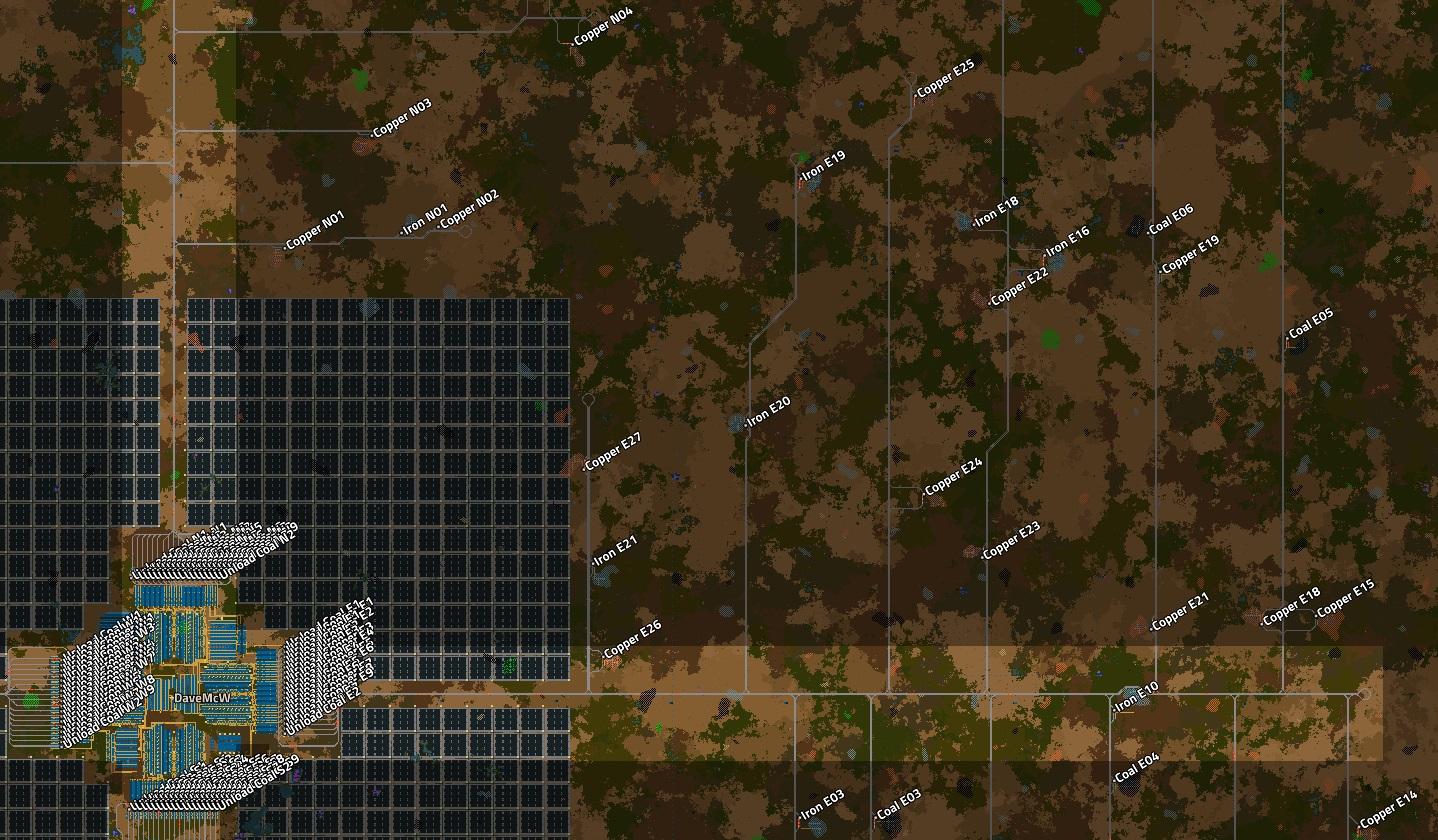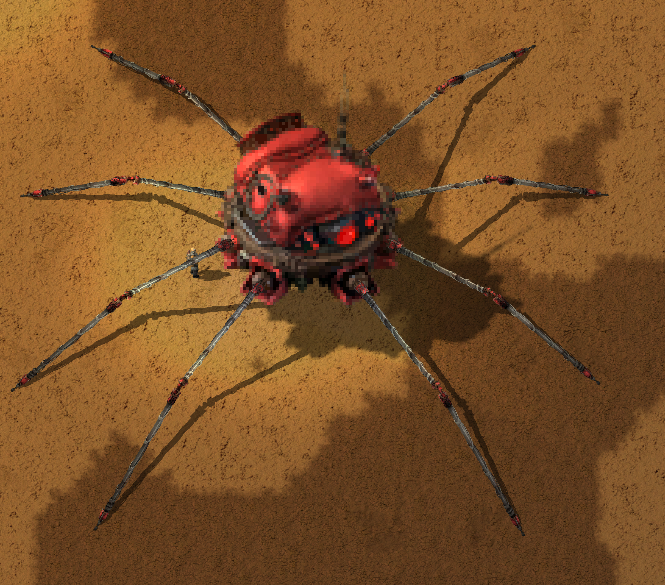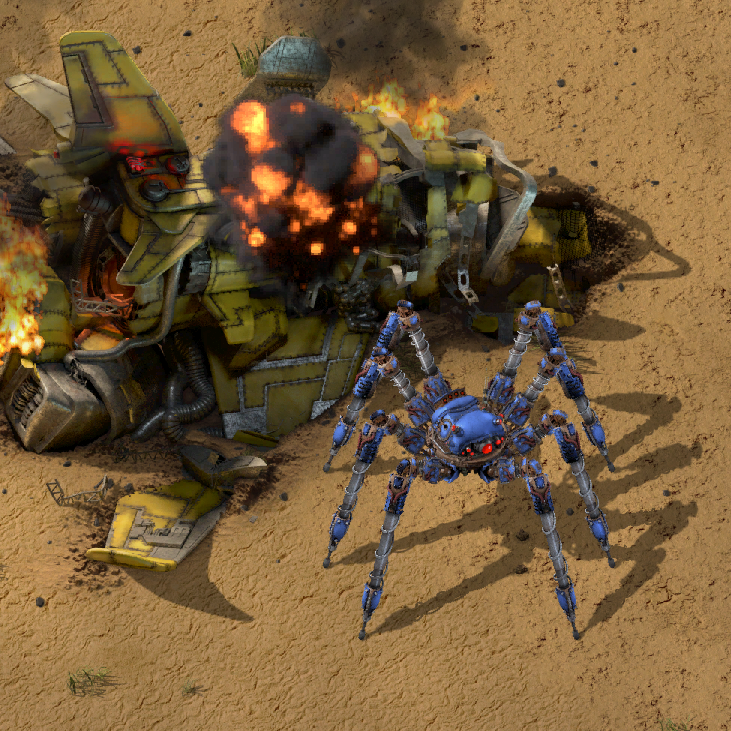# Alt-F4 #X Title of this post  2020-08-20

Welcome to this sample post, here we breafly describe how to use some advanced features of out templating configuration.

## Using Media

You can use media by just importing it in a regular markdown fascion

1. Images
• See using image.html below
• % include youtube.html id="28UzqVz1r24" %}
1. Dailymotion
• ![](https://www.dailymotion.com/video/x7tfyq3) or ![](https://dai.ly/x7tfyq3)
1. Vimeo
• ![](https://vimeo.com/263856289)
1. Spotify
• ![](https://open.spotify.com/track/4Dg5moVCTqxAb7Wr8Dq2T5)
1. SoundCloud
• ![](https://soundcloud.com/aviciiofficial/preview-avicii-vs-lenny)
1. General Audio
• ![](//www.soundhelix.com/examples/mp3/SoundHelix-Song-1.mp3)
1. Embeding a countdown
• % include countdown.html date="2021:01:01" %}
• Where date is the date in year:month:day:hour:minutes:seconds, UTC

## Using image.html

### Image only

Image embed parameters:

• src - Link
• alt - Alt-text
• caption - Text below the image
• href - High resolution version on click
• src-mob - Link for mobile version
• width - Max width
• height - Max height

### Example 1 - Caption + High res

% include image.html src='https://media.alt-f4.blog/ALTF4/13/base_new_map.jpg' alt='Map view of rail network, showing only an eighth of the outposts.' caption='Map view of rail network, showing just 27 of the 216 outposts.' href='https://media.alt-f4.blog/ALTF4/13/base_new_map.jpg' %}

### Example 2 - Simpler mobile

% include image.html src='https://media.alt-f4.blog/ALTF4/13/base_new_map.jpg' alt='Map view of rail network, showing only an eighth of the outposts.' caption='Map view of rail network, showing just 27 of the 216 outposts.' src-mob='https://img.apmcdn.org/768cb350c59023919f564341090e3eea4970388c/normal/5034f3-20180309-rick-astley.jpg' %}Map view of rail network, showing just 27 of the 216 outposts.

### Example 3 - All the things!

% include image.html src='https://media.alt-f4.blog/ALTF4/13/base_new_map.jpg' alt='Map view of rail network, showing only an eighth of the outposts.' caption='Map view of rail network, showing just 27 of the 216 outposts.' href='https://media.alt-f4.blog/ALTF4/13/base_new_map.jpg' src-mob='https://img.apmcdn.org/768cb350c59023919f564341090e3eea4970388c/normal/5034f3-20180309-rick-astley.jpg' width='500px' %}

## Using Math: MathJaxDr.MagPie

You can use MathJax to describe mathematical equasions and formulas. You can find full specification in MathJax Documentation but here are come samples

This code sample $a * b = c ^ b$ will transform into thes formula

$a * b = c ^ b$

You can go full in and make some like this

\begin{aligned} & \phi(x,y) = \phi \left(\sum_{i=1}^n x_ie_i, \sum_{j=1}^n y_je_j \right) = \sum_{i=1}^n \sum_{j=1}^n x_i y_j \phi(e_i, e_j) = \ & (x_1, \ldots, x_n) \left( \begin{array}{ccc} \phi(e_1, e_1) & \cdots & \phi(e_1, e_n) \ \vdots & \ddots & \vdots \ \phi(e_n, e_1) & \cdots & \phi(e_n, e_n) \end{array} \right) \left( \begin{array}{c} y_1 \ \vdots \ y_n \end{array} \right) \end{aligned}


Which will look like this

\begin{aligned} & \phi(x,y) = \phi \left(\sum_{i=1}^n x_ie_i, \sum_{j=1}^n y_je_j \right) = \sum_{i=1}^n \sum_{j=1}^n x_i y_j \phi(e_i, e_j) = \\ & (x_1, \ldots, x_n) \left( \begin{array}{ccc} \phi(e_1, e_1) & \cdots & \phi(e_1, e_n) \\ \vdots & \ddots & \vdots \\ \phi(e_n, e_1) & \cdots & \phi(e_n, e_n) \end{array} \right) \left( \begin{array}{c} y_1 \\ \vdots \\ y_n \end{array} \right) \end{aligned}

You can preview your formulas here

## Juxtapose

### Imagesevery subsequent gallery MUST have offset parameter set to a sum of all images in previous galleries

### ToolTip / info popup

This is manual tooltip text after tooltip

this is embedded tooltip use which ever you like more